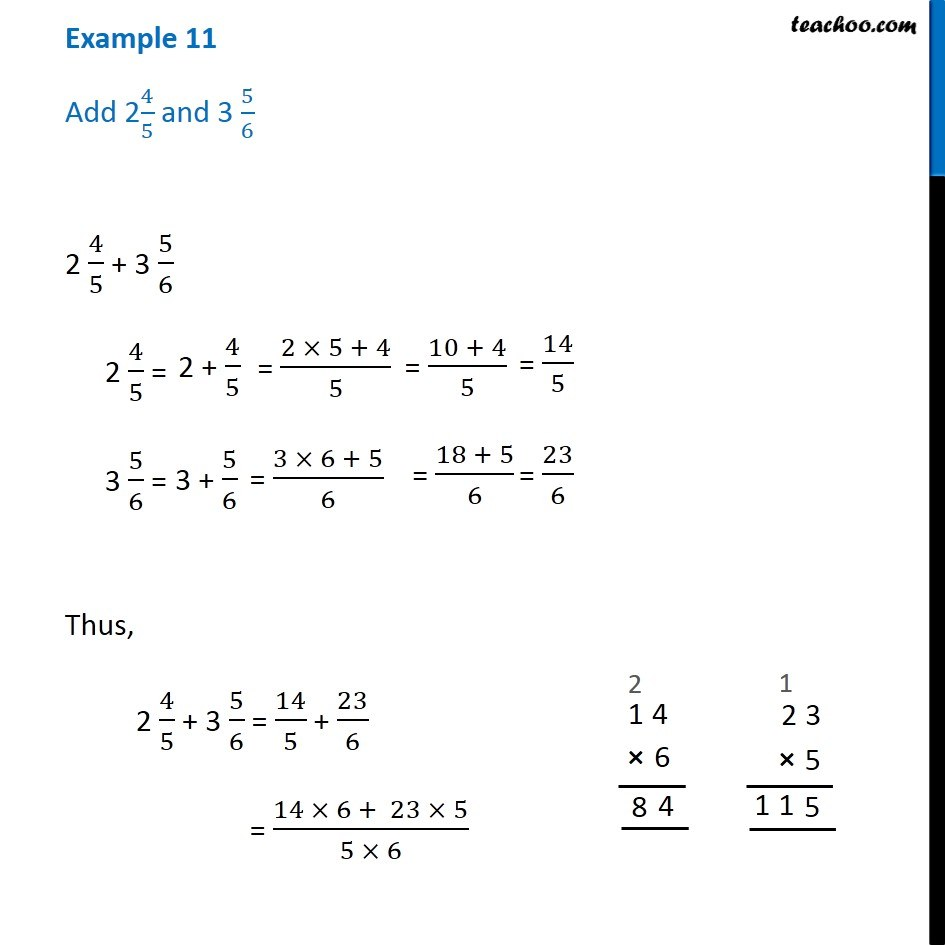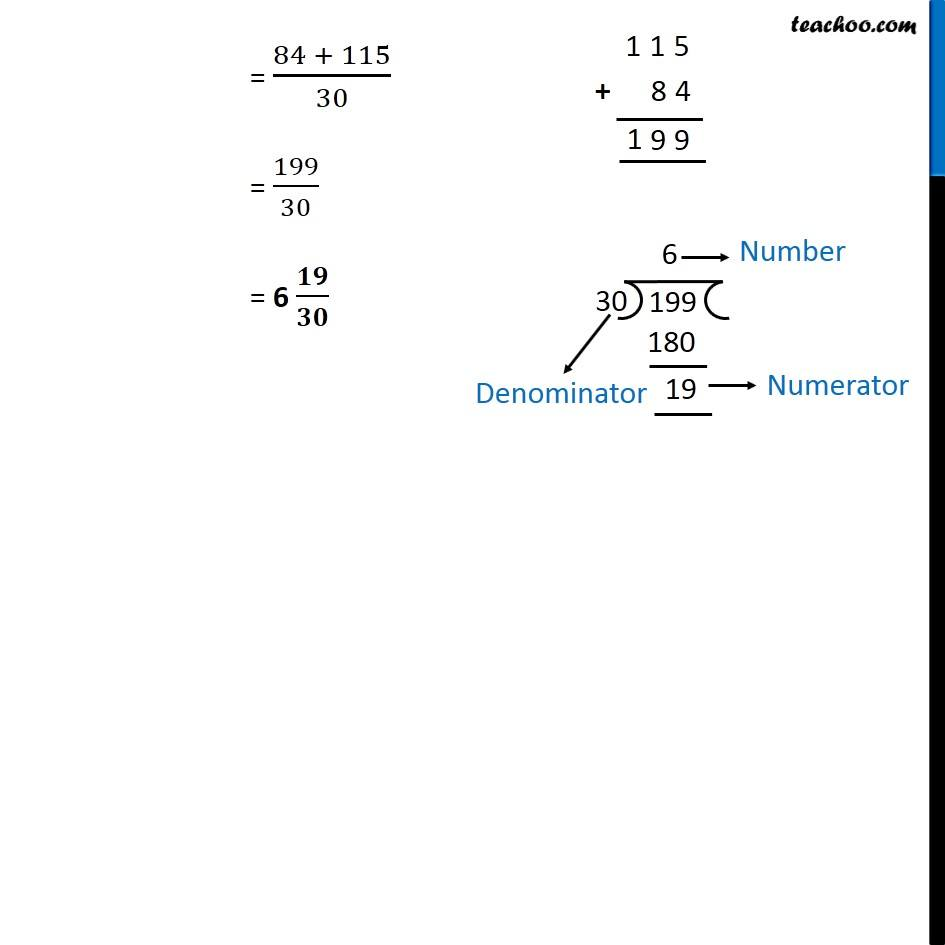Addition and Subtraction of Mixed Fractions

Chapter 7 Class 6 Fractions
Concept wiseIntroducing your new favourite teacher - Teachoo Black, at only ₹83 per month

### Transcript

Example 11 Add 24/5 and 3 5/6 2 4/5 + 3 5/6 2 4/5 = 3 5/6 = Thus, 2 4/5 + 3 5/6 = 14/5 + 23/6 = (14 × 6 + 23 × 5)/(5 × 6) = (84 + 115)/30 = 199/30 = 6 𝟏𝟗/𝟑𝟎